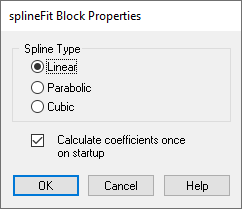## splineFit

Block Category: Matrix Operation

Description: The splineFit block calculates a spline fit to two vectors of X,Y data, and returns the resulting curves with the second block input as the independent variable. The top two inputs accept columns of X- and Y-coordinates, respectively. The bottom input takes the input signal. Output is the modified input signal.Calculate coefficients once on startup: Calculates the polynomial fit once at start of the simulation, which is fine for static data sets. The fit algorithm is numerically costly, so it should only be run if needed.

#### Spline Type

Cubic: Calculates the piece-wise polynomial of degree 3.

Linear: Calculates the piece-wise polynomial of degree 1.

Parabolic: Calculates the piece-wise polynomial of degree 2.